# Evolutionary Rates & Selection Mode (Mr.Bayes)

#### 2022-11-03

This vignette explains how to extract evolutionary rate parameters estimated from relaxed clock Bayesian inference analyses produced by Mr. Bayes. It also shows how to use evolutionary rate based inference of selection mode (strength) adapted to clock-based rates, as introduced by Simões and Pierce (2021). See the sister vignette “Evolutionary Rates & Selection Mode (BEAST2)” for an equivalent workflow using output data produced by BEAST2

library(EvoPhylo)

## Evolutionary Rates Statistics and Plots

In this section, we will extract evolutionary rate parameters from each node from a Bayesian clock (time-calibrated) summary tree. The functions below will store them in a data frame, produce summary statistics tables, and create different plots showing how rates are distributed across morphological partitions and clades.

### 1. Get rates from the clock tree and create a rate table

First, import a Bayesian clock tree using treeio’s function read.mrbayes() (= read.beast()).

## Import summary tree with three clock partitions produced by
## Mr. Bayes (.t or .tre files) from your local directory

tree3p <- treeio::read.mrbayes("Tree3p.t")

Below, we use the example Mr.Bayes single multi-clock tree with 3 morphological partitions tree3p from Simões and Pierce (2021) that accompanies EvoPhylo.

data(tree3p)

Subsequently, using get_clockrate_table_MrBayes(), users can extract mean or median rate values for each node in the summary tree that were annotated by Mr. Bayes when creating the summary tree with Mr. Bayes “sumt” command. These mean or median rate values are calculated by Mr. Bayes taking into account all trees from the posterior sample. This works for any summary tree produced by Mr. Bayes: a majority rule consensus or the fully resolved maximum compatible tree (the latter is used in the examples here).

Please note that analyses must have reached the stationarity phase and independent runs converging for the summary statistics in each node to be meaningful summaries of the posterior sample.

## Get table of clock rates with summary stats for each node in
## the tree for each relaxed clock partition (3 partitions in this tree file)
RateTable_Means_3p <- get_clockrate_table_MrBayes(tree3p, summary = "mean")

### 2. Export the rate table and plot tree with node values

Once a rate table has been obtained from Mr. Bayes it is necessary to export it. This is a necessary step to subsequently open the rate table spreadsheet locally (e.g., using Microsoft Office Excel) and customize the table with clade names associated with with each node in the tree for downstream analyses. Note that the root node may include “NA” for rate value, so it must be removed from the rate table.

## Export the rate tables (using Mr. Bayes example with 3 partitions)
write.csv(RateTable_Means_3p, file = "RateTable_Means_3p.csv")

## Plot tree node labels to customize clade names

To visualize the node values in the tree, you can use ggtree().

## Plot tree node labels
library(ggtree)
tree_nodes <- ggtree(tree3p, branch.length = "none", size = 0.05) +
geom_tiplab(size = 2, linesize = 0.01, color = "black", offset = 0.5) +
geom_label(aes(label = node), size = 2, color="purple")
tree_nodes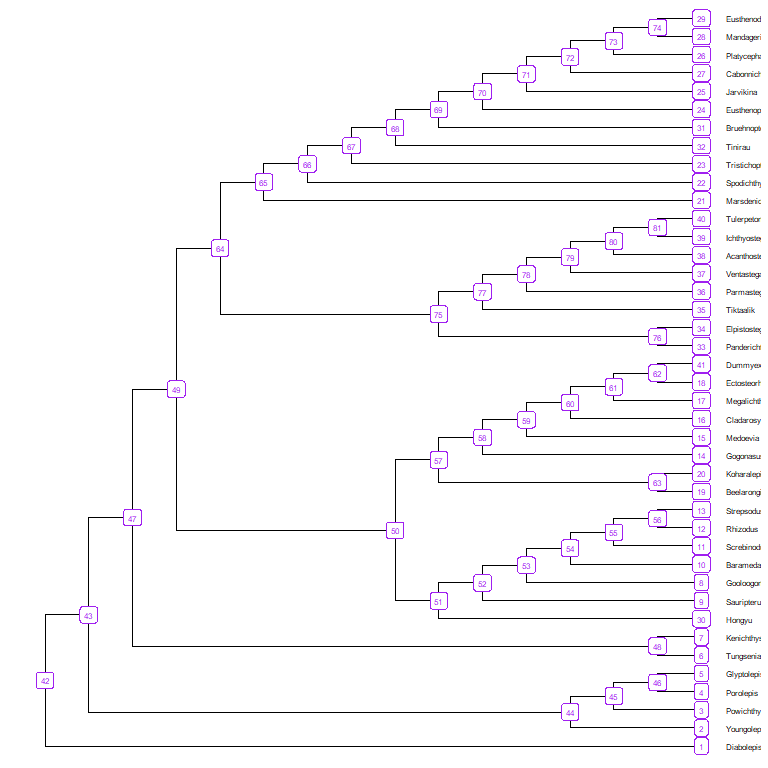## Save your plot to your working directory as a PDF
ggplot2::ggsave("Tree_nodes.pdf", width = 10, height = 10)

### 3. Import rate table with custom clade memberships

## Import rate table with clade membership (new "clade" column added)
RateTable_Means_3p_Clades <- read.csv("RateTable_Means_3p_Clades.csv", header = TRUE)

Below, we use the rate table with clade membership RateTable_Means_3p_Clades that accompanies EvoPhylo.

data(RateTable_Means_3p_Clades)

##         clade nodes   rates1   rates2   rates3
## 1 Dipnomorpha     1 0.943696 0.981486 1.006164
## 2 Dipnomorpha     2 1.065326 0.772074 0.913194
## 3 Dipnomorpha     3 1.182460 0.656872 0.813618
## 4 Dipnomorpha     4 1.229767 0.523709 0.722519
## 5 Dipnomorpha     5 1.230564 0.517773 0.720479
## 6       Other     6 0.658855 0.717277 0.663950

### 4. Get summary stats for each clade/clock partition

Obtain summary statistics table and plots for each clade by clock partition using clockrate_summary(). Supplying a file path to file save the output to that file.

## Get summary statistics table for each clade by clock
file = "Sum_RateTable_Means_3p.csv")
Rate table summary statistics
clade clock n mean sd min Q1 median Q3 max
Dipnomorpha 1 8 1.10 0.11 0.94 1.02 1.10 1.19 1.23
Elpisostegalia 1 14 1.61 0.22 1.13 1.45 1.68 1.80 1.81
Osteolepididae 1 11 0.63 0.26 0.16 0.44 0.81 0.84 0.87
Rhizodontidae 1 14 0.57 0.30 0.03 0.33 0.67 0.83 0.89
Tristichopteridae 1 21 0.71 0.04 0.61 0.69 0.72 0.74 0.78
Other 1 11 0.89 0.36 0.54 0.69 0.78 0.94 1.81
Dipnomorpha 2 8 0.75 0.18 0.52 0.62 0.75 0.89 0.98
Elpisostegalia 2 14 1.36 0.10 1.03 1.36 1.38 1.41 1.42
Osteolepididae 2 11 0.34 0.15 0.07 0.28 0.38 0.45 0.53
Rhizodontidae 2 14 0.33 0.18 0.02 0.17 0.38 0.44 0.56
Tristichopteridae 2 21 0.34 0.06 0.27 0.32 0.33 0.33 0.55
Other 2 11 0.75 0.25 0.39 0.61 0.72 0.78 1.35
Dipnomorpha 3 8 0.87 0.11 0.72 0.79 0.89 0.95 1.01
Elpisostegalia 3 14 0.83 0.16 0.63 0.67 0.89 0.99 1.00
Osteolepididae 3 11 0.32 0.13 0.07 0.27 0.33 0.42 0.49
Rhizodontidae 3 14 0.32 0.17 0.02 0.21 0.40 0.43 0.52
Tristichopteridae 3 21 0.52 0.08 0.37 0.44 0.54 0.59 0.64
Other 3 11 0.73 0.17 0.47 0.64 0.70 0.81 1.00

### 5. Plot rates by clock partition and clade

Plot distributions of rates by clock partition and clade with clockrate_dens_plot().

## Overlapping plots
nrow = 1, scales = "fixed")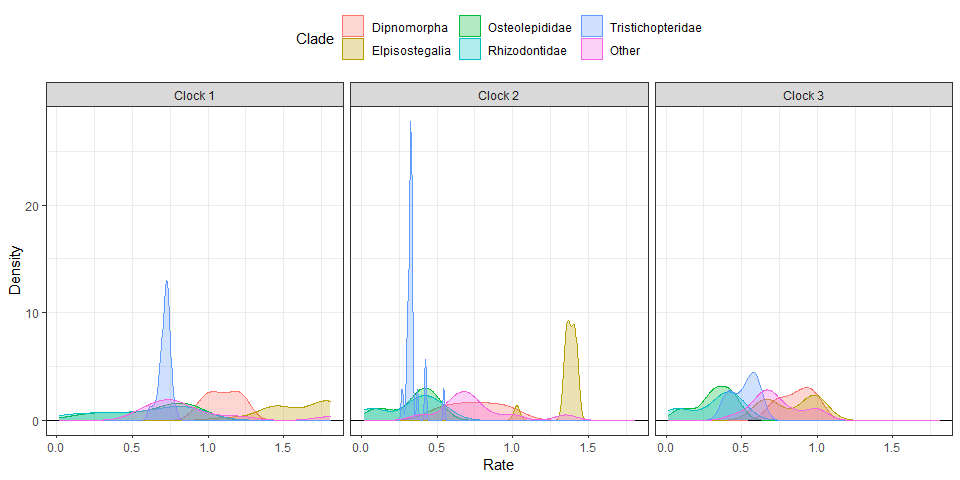Sometimes using stacked plots provides a better visualization as it avoids overlapping distributions.

## Stacked plots
nrow = 1, scales = "fixed")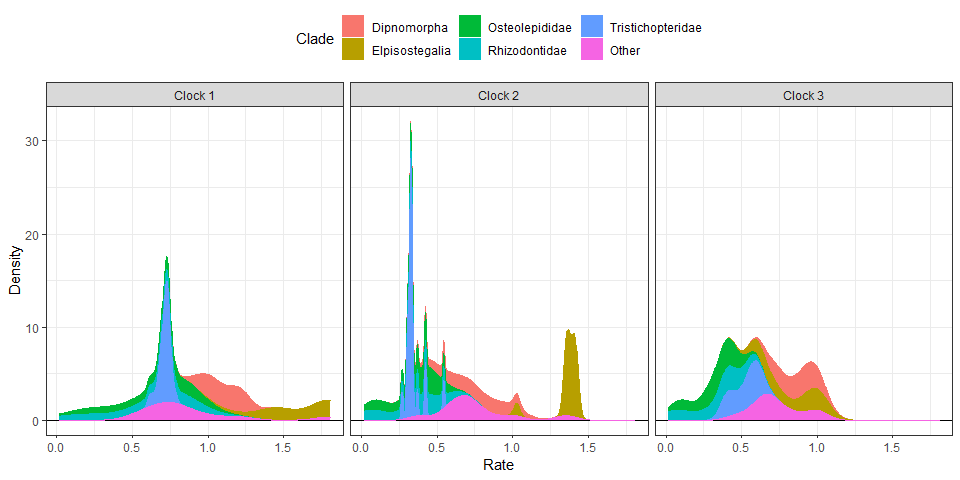It is also possible to append extra layers using ggplot2 function, such as for changing the color scale. Below, we change the color scale to be the Viridis scale.

## Stacked plots with viridis color scale
nrow = 1, scales = "fixed") +
ggplot2::scale_color_viridis_d() +
ggplot2::scale_fill_viridis_d()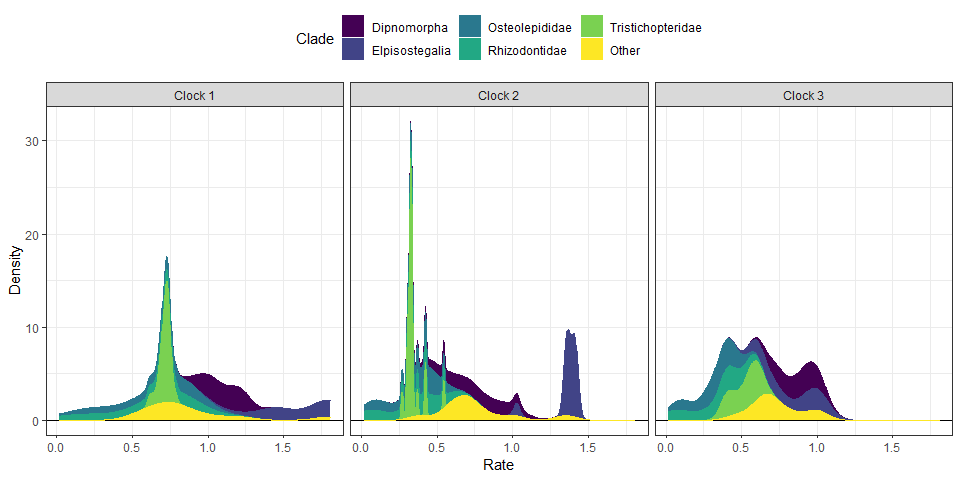### 6. Rate linear models

We can also plot linear model regressions between rates from two or more clocks with clockrate_reg_plot().

## Plot regressions of rates from two clocks
p12 <- clockrate_reg_plot(RateTable_Means_3p_Clades, clock_x = 1, clock_y = 2)
p13 <- clockrate_reg_plot(RateTable_Means_3p_Clades, clock_x = 1, clock_y = 3)
p23 <- clockrate_reg_plot(RateTable_Means_3p_Clades, clock_x = 2, clock_y = 3)

library(patchwork) #for combining plots
p12 + p13 + p23 + plot_layout(ncol = 2)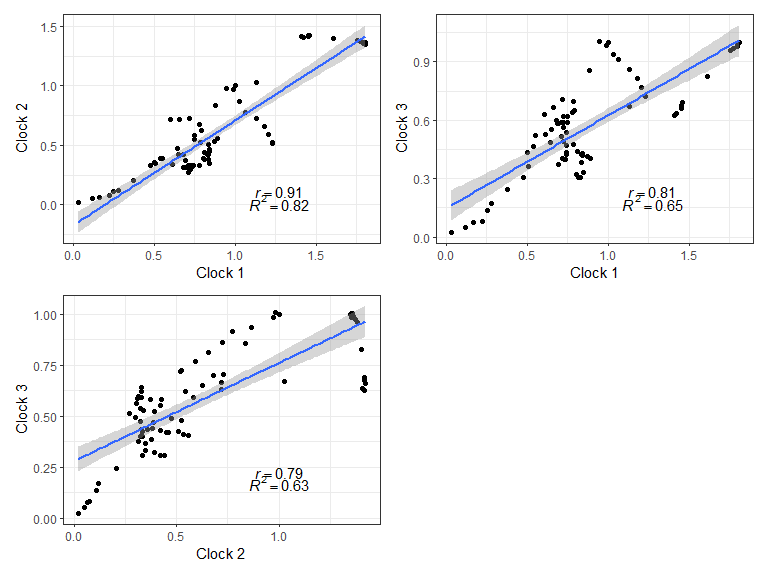## Save your plot to your working directory as a PDF
ggplot2::ggsave("Plot_regs.pdf", width = 8, height = 8)

### ADDENDUM: Example using rates from single clock analysis (no partitioning)

You can also explore clock rates for summary trees including a single clock shared among all character partitions (or an unpartitioned analysis):

## Import summary tree with a single clock partitions produced by
## Mr. Bayes (.t or .tre files) from examples directory
tree1p <- treeio::read.mrbayes("Tree1p.t")

Below, we use the example tree tree1p that accompanies EvoPhylo.

data(tree1p)

Then, get table of clock rates with summary stats for each node in the tree for each relaxed clock partition.

RateTable_Means_1p <- get_clockrate_table_MrBayes(tree1p, summary = "mean")

Then proceed with the analysis, as in the case with multiple clocks.

## Export the rate tables
write.csv(RateTable_Means_1p, file = "RateTable_Means1.csv")

RateTable_Means_1p_Clades <- read.csv("RateTable_Means1_Clades.csv", header = TRUE)
#Below, we use the rate table with clade membership RateTable_Means_1p_Clades that accompanies EvoPhylo.
data(RateTable_Means_1p_Clades)
## Get summary statistics table for each clade by clock
file = "Sum_RateTable_Medians1.csv")
Rate table summary statistics
clade n mean sd min Q1 median Q3 max
Dipnomorpha 8 0.57 0.28 0.22 0.37 0.54 0.78 0.95
Elpisostegalia 14 0.91 0.25 0.44 0.77 0.85 1.03 1.35
Osteolepididae 11 0.23 0.10 0.03 0.18 0.23 0.30 0.38
Rhizodontidae 14 0.18 0.15 0.00 0.04 0.20 0.29 0.42
Tristichopteridae 21 0.39 0.43 0.05 0.11 0.19 0.34 1.32
Other 11 0.41 0.26 0.20 0.25 0.28 0.45 1.00
## Stacked plots with viridis color scale
nrow = 1, scales = "fixed") +
ggplot2::scale_color_viridis_d() +
ggplot2::scale_fill_viridis_d()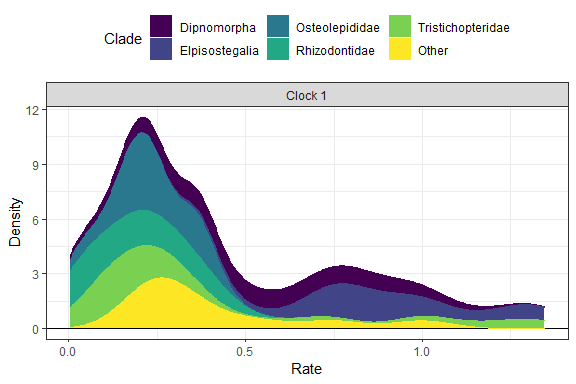## Selection Mode

In this section, we will use evolutionary rate based inference of selection mode, as first introduced by Baker et al. (2016) for continuous traits, and later adapted to clock-based rates by Simões and Pierce (2021).

### 1. Import combined log file from all runs.

This is produced by using combine_log(). The first argument passed to combine_log() should be a path to the folder containing the log files to be imported and combined.

## Import all log (.p) files from all runs and combine them, with burn-in = 25%
## and downsampling to 2.5k trees in each log file
posterior3p <- combine_log("LogFiles3p", burnin = 0.25, downsample = 1000)

Below, we use the posterior dataset posterior3p that accompanies EvoPhylo.

data(posterior3p)

## Show first 10 lines of combined log file
head(posterior3p, 10)

###2. Check background rates distribution and if they need transformation

The output includes histograms showing the data distribution before and after data transformation for comparisons.

library(ggplot2)
B1 <- plot_back_rates (type = "MrBayes", posterior3p, clock = 1,
trans = "log10", size = 10, quantile = 1)
B1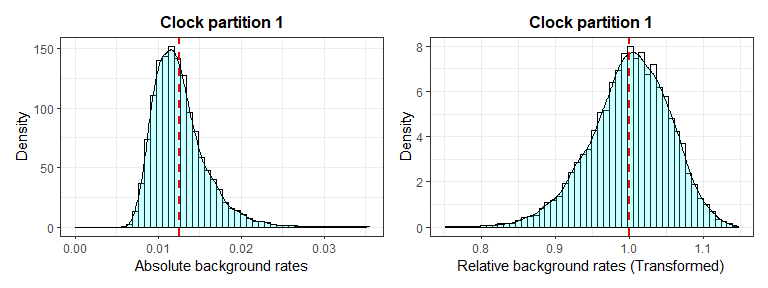B2 <- plot_back_rates (type = "MrBayes", posterior3p, clock = 2,
trans = "log10", size = 10, quantile = 1)
B2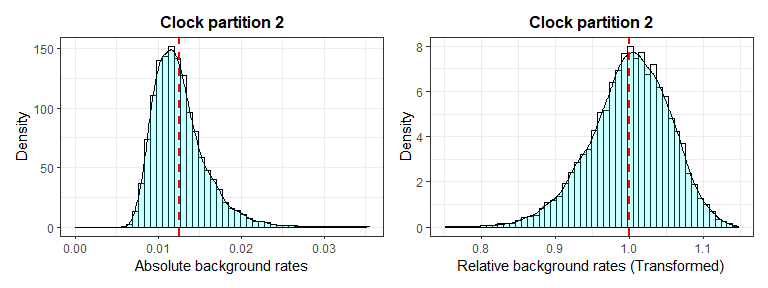B3 <- plot_back_rates (type = "MrBayes", posterior3p, clock = 3,
trans = "log10", size = 10, quantile = 1)
B3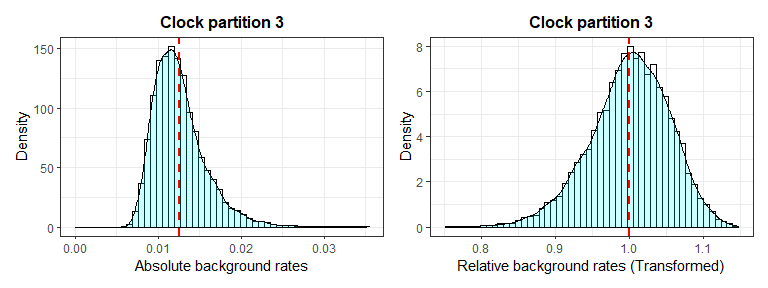### 3. Plot selection gradient on the summary tree

Using different thresholds, identify the selection mode across branches in the tree for each clock partition with plot_treerates_sgn().

Users must indicate the type of output file (between Mr. Bayes and BEAST2) and whether they would like to log transform the background rate to meet assumptions of normally distributed data, based on the results obtained from plot_back_rates. Users should also indicate in “clock” the number of the clock partition they would like to plot rates from and the desired significance threshold to interpret branch rates (we recommend number of standard deviations around the mean of background rates).Finally, a series of arguments enable users to customize the geological timescale to add to the tree.

## Plot tree using various thresholds for clock partition 1
A1 <- plot_treerates_sgn(
type = "MrBayes", trans = "none",                            #Indicates software name output and type of transformation
tree3p, posterior3p,                                         #Summary tree and posterior files
clock = 1,                                                   #Show rates for clock partition 1
summary = "mean",                                            #sets summary stats to get from summary tree nodes
branch_size = 1.5, tip_size = 3,                             #sets size for tree elements
xlim = c(-450, -260), nbreaks = 8, geo_size = list(3, 3),    #sets limits and breaks for geoscale
threshold = c("1 SD", "3 SD"))                               #sets threshold for selection mode
A1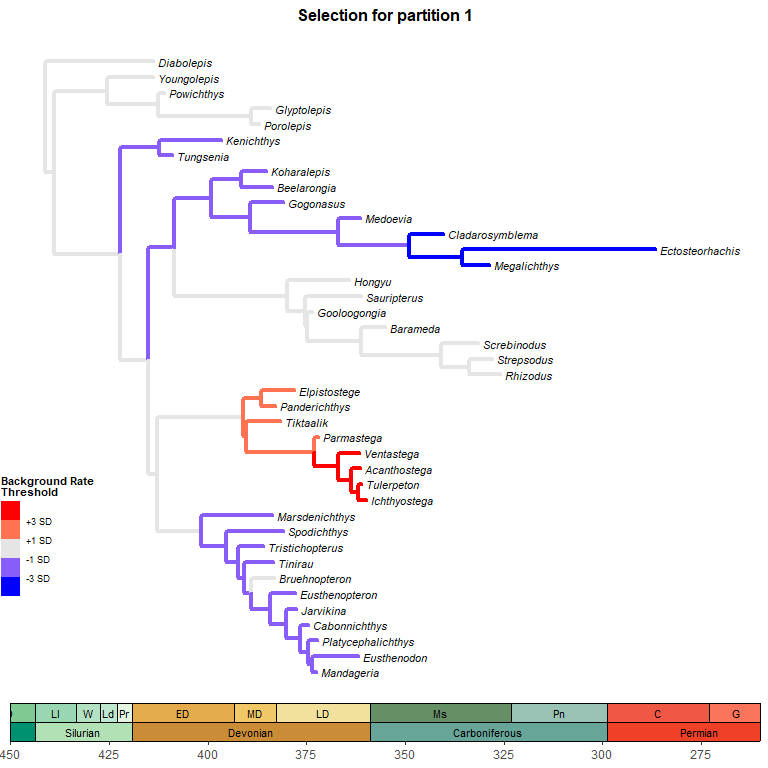Plot tree using various thresholds for the other clock partitions and combine them.

## Plot tree using various thresholds for other clock partition and combine them
A2 <- plot_treerates_sgn(
type = "MrBayes", trans = "none",                            #Indicates software name output and type of transformation
tree3p, posterior3p,                                         #Summary tree and posterior files
clock = 2,                                                   #Show rates for clock partition 1
summary = "mean",                                            #sets summary stats to get from summary tree nodes
branch_size = 1.5, tip_size = 3,                             #sets size for tree elements
xlim = c(-450, -260), nbreaks = 8, geo_size = list(3, 3),    #sets limits and breaks for geoscale
threshold = c("1 SD", "3 SD"))                               #sets threshold for selection mode

A3 <- plot_treerates_sgn(
type = "MrBayes", trans = "none",                            #Indicates software name output and type of transformation
tree3p, posterior3p,                                         #Summary tree and posterior files
clock = 3,                                                   #Show rates for clock partition 1
summary = "mean",                                            #sets summary stats to get from summary tree nodes
branch_size = 1.5, tip_size = 3,                             #sets size for tree elements
xlim = c(-450, -260), nbreaks = 8, geo_size = list(3, 3),    #sets limits and breaks for geoscale
threshold = c("1 SD", "3 SD"))                               #sets threshold for selection mode

library(patchwork)
A1 + A2 + A3 + plot_layout(nrow = 1)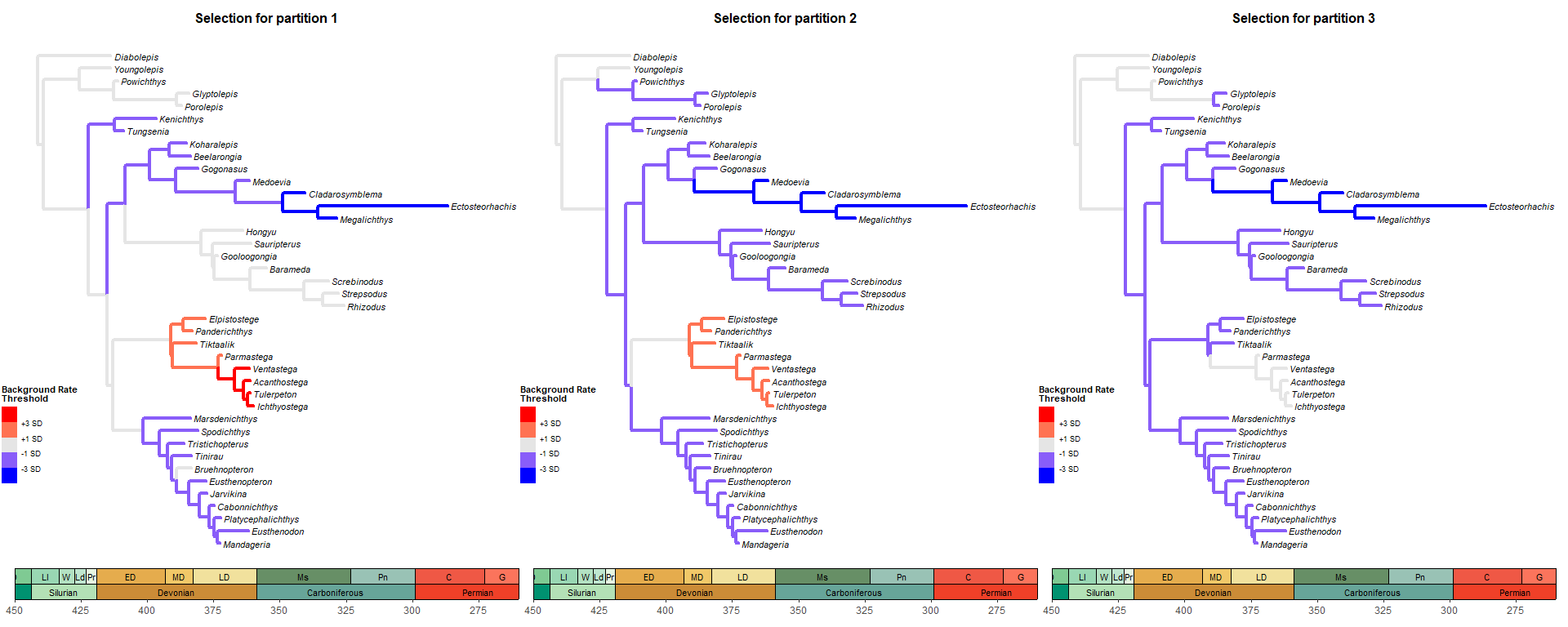## Save your plot to your working directory as a PDF
ggplot2::ggsave("Tree_Sel_3p.pdf", width = 20, height = 8)

### 4. Pairwise t-tests of rate values among clades

The function get_pwt_rates_MrBayes() complements the functionality of plot_treerates_sgn by producing a table of pairwise t-tests for differences between the mean background rate in the posterior and the absolute rate for each summary tree branches Should be used only for normally distributed data in which a CI=0.95 is considered a good threshold. In many cases, however, using multiple standard deviations as outputted using plot_treerates_sgn provides a more robust test of whether branch rates are significantly different from background rates.

## Import rate table with clade membership
RateTable_Means_3p_Clades <- read.csv("RateTable_Means_Clades.csv", header = TRUE)

Below, we use the rate table with clade membership RateTable_Means_3p_Clades that accompanies EvoPhylo.

data(RateTable_Means_3p_Clades)

4.2. Get and export table of pairwise t-tests

## Get table of pairwise t-tests for difference between the posterior
## mean and the rate for each tree node

## Show first 10 lines of table
head(RateSign_Tests, 10)
Combined log file
clade nodes clock relative.rate.mean absolute.rate.mean p.value
Dipnomorpha 1 1 0.943696 0.0118443 0
Dipnomorpha 2 1 1.065326 0.0133709 0
Dipnomorpha 3 1 1.182460 0.0148411 0
Dipnomorpha 4 1 1.229767 0.0154348 0
Dipnomorpha 5 1 1.230564 0.0154448 0
Other 6 1 0.658855 0.0082693 0
Other 7 1 0.603090 0.0075694 0
Osteolepididae 8 1 0.843373 0.0105852 0
Osteolepididae 9 1 0.872012 0.0109446 0
Osteolepididae 10 1 0.811473 0.0101848 0
## Export the table
write.csv(RateSign_Tests, file = "RateSign_Tests.csv")